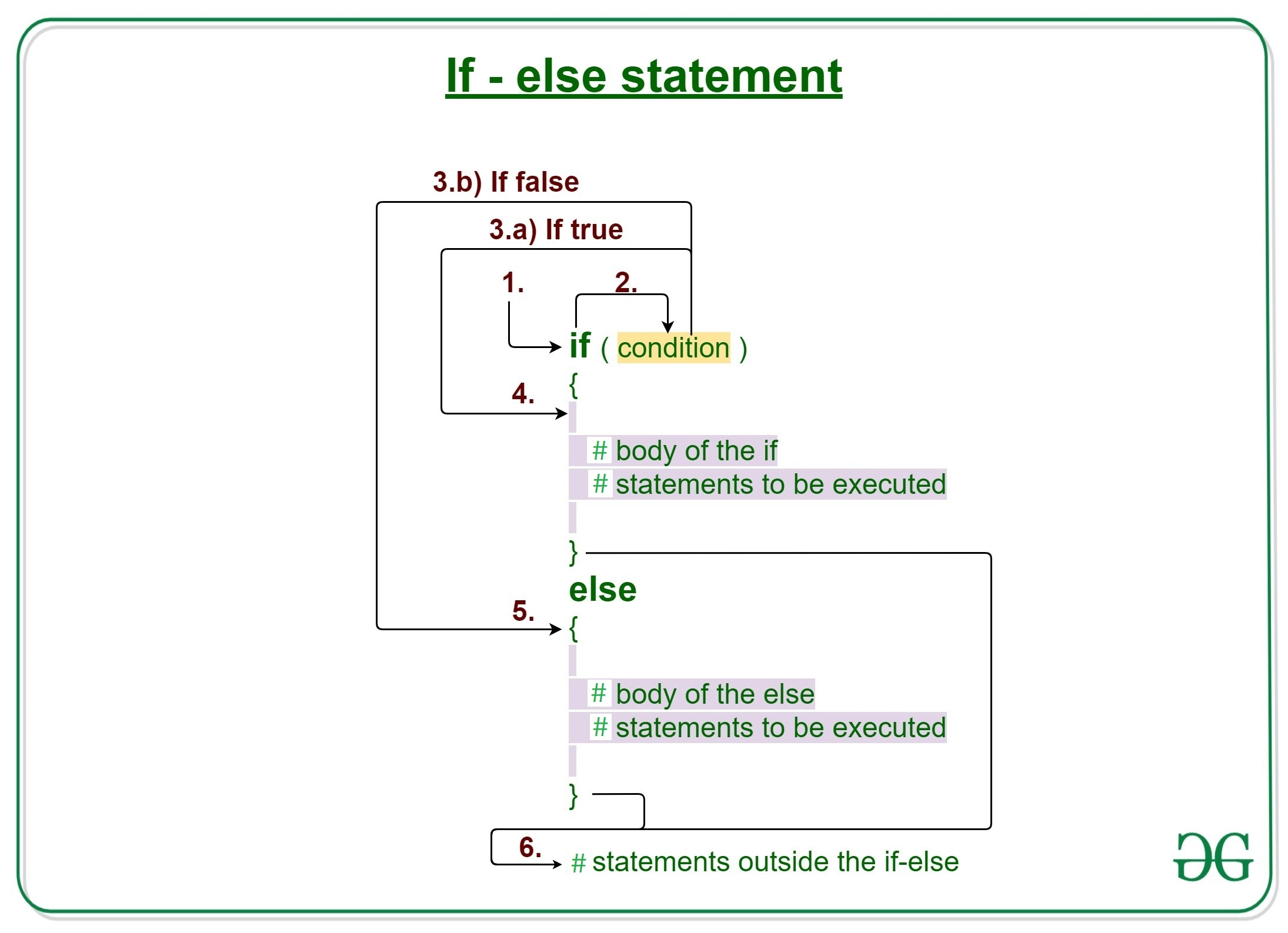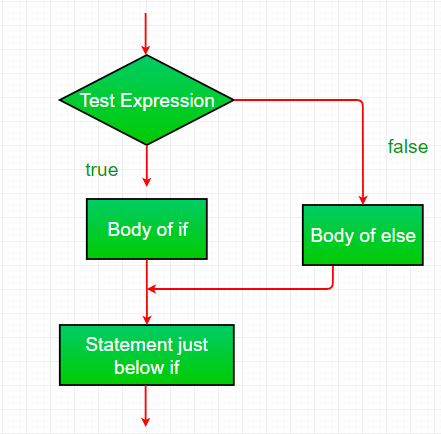# R – if-else statement

• Last Updated : 18 Oct, 2021

The if-statement in Programming Language alone tells us that if a condition is true it will execute a block of statements and if the condition is false it won’t. But what if we want to do something else if the condition is false. Here comes the R else statement. We can use the else statement with the if statement to execute a block of code when the condition is false.

### Syntax of if-else statement in R Language:

```if (condition)
{
// Executes this block if
// condition is true
} else
{
// Executes this block if
// condition is false
}```## Working of if-else statement in R Programming

• Control falls into the if block.
• The flow jumps to Condition.
• Condition is tested.
• If Condition yields true, goto Step 4.
• If Condition yields false, goto Step 5.
• The if-block or the body inside the if is executed.
• The else block or the body inside the else is executed.
• Flow exits the if-else block.

### Flowchart if-else statement in R:Example 1:

## R

 `x <- 5`` ` `# Check value is less than or greater than 10``if``(x > 10)``{``    ``print``(``paste``(x, ``"is greater than 10"``))``} ``else``{``    ``print``(``paste``(x, ``"is less than 10"``))``}`

Output:

` "5 is less than 10"`

Here in the above code, Firstly, x is initialized to 5, then if-condition is checked(x > 10), and it yields false. Flow enters the else block and prints the statement “5 is less than 10”.

Example 2:

## R

 `x <- 5` `# Check if value is equal to 10``if``(x == 10)``{``    ``print``(``paste``(x, ``"is equal to 10"``))``} ``else``{``    ``print``(``paste``(x, ``"is not equal to 10"``))``}`

Output:

` "5 is not equal to 10" `

## Nested if-else statement in R

The if-else statements can be nested together to form a group of statements and evaluate expressions based on the conditions one by one, beginning from the outer condition to the inner one by one respectively. An if-else statement within another if-else statement better justifies the definition.

### Syntax:

```if(condition1){
# execute only if condition 1 satisfies
if(condition 2){
# execute if both condition 1 and 2 satisfy
}
}else{
}```

Example:

## R

 `# creating values``var1 <- 6``var2 <- 5``var3 <- -4` `# checking if-else if ladder``if``(var1 > 10 || var2 < 5){``print``(``"condition1"``)``}``else``{``if``(var1 <4 ){``    ``print``(``"condition2"``)``}``else``{``    ``if``(var2>10){``    ``print``(``"condition3"``)``    ``}``    ``else``{``    ``print``(``"condition4"``)``    ``}``}``}`

Output:

` "condition4"`

My Personal Notes arrow_drop_up